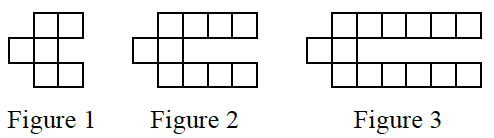### Home > CC4 > Chapter 1 > Lesson 1.1.2 > Problem1-15

1-15.Examine the tile pattern shown at right. 1-15 HW eTool (CPM).  Homework Help ✎

1. On graph paper, draw Figure 4.

2. How many tiles will Figure 10 have? How do you know?

Use the eTool to highlight the new tiles in each figure with a different color. How many new tiles are added each time?

To find the number of tiles in Figure 10 you can make a table and extend it to Figure 10, write an equation and let $x = 10$, or think about what figure $10$ would look like. The two tiles in the middle row are always there. Notice how many pairs of tiles are in each arm of the figure. Sketch figure 10 and determine the number of tiles.

Use the eTool below to examine and draw the tile pattern.
Click the link at right for a full version of the eTool: Int1 1-15 HW eTool